#### You may also like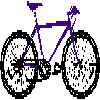Four vehicles travelled on a road. What can you deduce from the times that they met?### Intersections

Change one equation in this pair of simultaneous equations very slightly and there is a big change in the solution. Why?### Graphical Triangle

What is the area of the triangle formed by these three lines?

# Perpendicular Lines

##### Age 14 to 16Challenge Level

We received correct solutions from Ash and Lucy, two students at Tiffin Girls' School. Well done to you both.
Ash sent us this correct solution:

The gradient of the first line must be the negative reciprocal of the gradient of the other line:
if you multiply the two gradients you always get -1.

For example:

Take a random gradient, say $\frac{4}{7}$
The negative reciprocal gradient will be $\frac{-7}{4}$

Make up two equations with these gradients, say
$y =\frac{4x}{7}$ and $y =\frac{-7x}{4}$

Draw them on a grid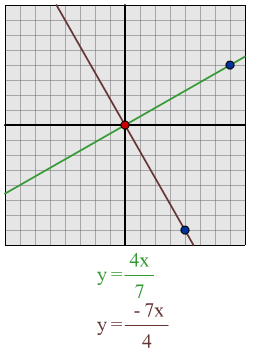You get perpendicular lines.

Lucy recorded how she worked through this problem:

$y = x$ is perpendicular to $y = -x$

$y = x$ is perpendicular to $y = -x + 2$
(the y-intercept doesn't affect the gradient of the line)

$y = 2x$ is perpendicular to $y = -x/2$

$y = -3x$ is perpendicular to $y = x/3$
(to make a line perpendicular you need to invert the gradient, or take the reciprocal, and change the sign)

$y = -2x$ is perpendicular to $y = x/2$

I can see a pattern here: when the two gradients of perpendicular lines are multiplied together they give -1, and the y-intercept does not affect if the line is perpendicular or not.

I will now try to work out what the perpendicular line of some other lines will be using this formula:

$y = 7x - 3$:

Using my formula I predict that a line which is perpendicular to this line will be $y = -x/7 - 3$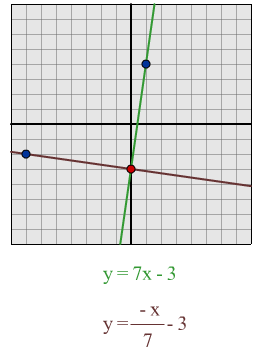When I tested the lines out, I found that the formula had worked.

$y = x/3 + 4$:

Using my formula I predict that a line which is perpendicular to this line will be $y = -3x + 4$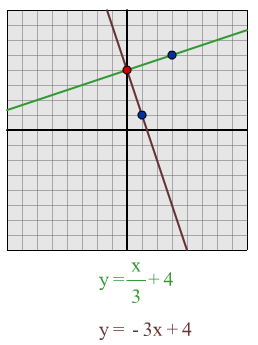Having drawn out the lines I found that the formula worked and the lines were perpendicular.

$y = -7x/3 + 2$:

I predict that a line which is perpendicular to this line will be $y = 3x/7$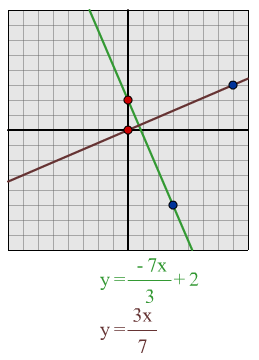From drawing out these lines I can see that they are perpendicular.